Function Repository Resource:

# BinListsBy

Bin data into lists based on applying a function to each item

Contributed by: Sander Huisman
 ResourceFunction["BinListsBy"][{x1,x2,…},{f,xmin,xmax}] gives lists of the elements xi for which the values of f[xi] lie in bins from xmin to xmax with unit width. ResourceFunction["BinListsBy"][{x1,x2,…},{f,xmin,xmax,Δx}] gives lists of the elements xi for which the values of f[xi] lie in bins from xmin to xmax with width Δx. ResourceFunction["BinListsBy"][{x1,x2,…},binspec1,binspec2,…] gives an array of lists of the elements xi for which each index is determined by applying fi of the binning specifications binspeci to the value xi.

## Details and Options

ResourceFunction["BinListsBy"] drops those elements for which the function evaluates to values that are outside the binning specification.
ResourceFunction["BinListsBy"] drops those elements for which the function evaluates to complex numbers or to non-numerical data.
Within each bin, elements appear in the same order as in the original data.
ResourceFunction["BinListsBy"] places elements in bin i when their function values satisfy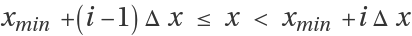.

## Examples

### Basic Examples (4)

Bin some data based only on the first element of each list:

 In:=Out=Bin a list of data based on the string length of each item:

 In:=Out=Bin some data based on the total of each list:

 In:=Out=Bin some data with some "auxiliary" data:

 In:=Out=### Scope (3)

Perform a 2-dimensional binning based on the value of the first element, and the string length of the last element:

 In:=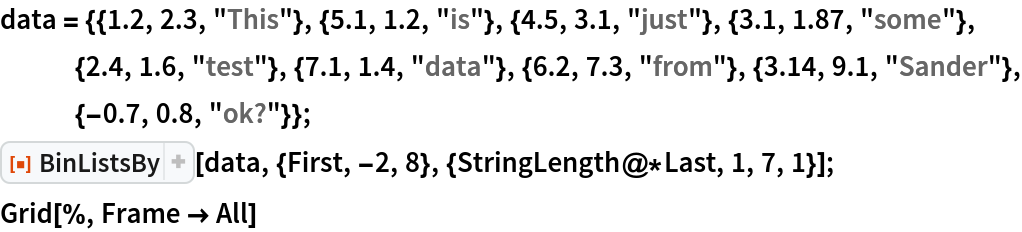Out=Perform a 3-dimensional binning based on the value of the first element, the value of the middle element, and the length of the last element:

 In:=Out=The lists do not need to have the same length, structure, or type, allowing to bin data with auxiliary data:

 In:=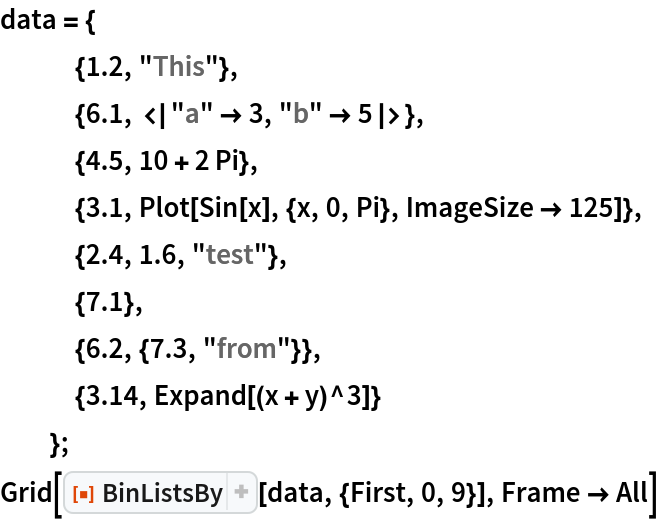Out=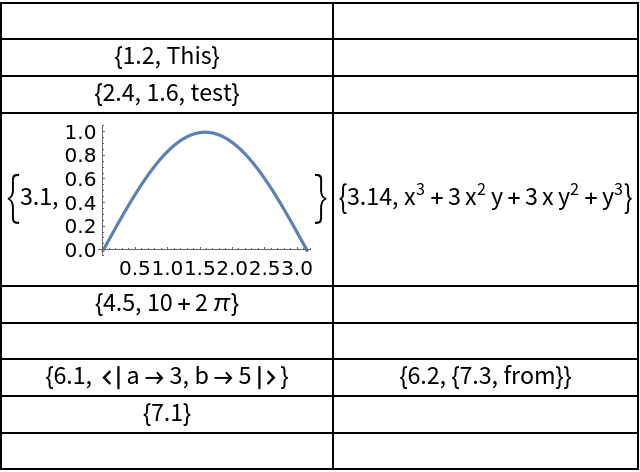### Applications (5)

Bin some data based on the number of unique letters in each string:

 In:=Out=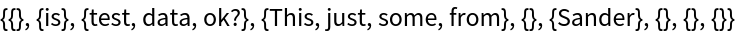Bin complex numbers based on their imaginary and real parts:

 In:=Out=Bin some strings based on their first letter:

 In:=Out=Bin some person entities based on their birth century:

 In:=Out=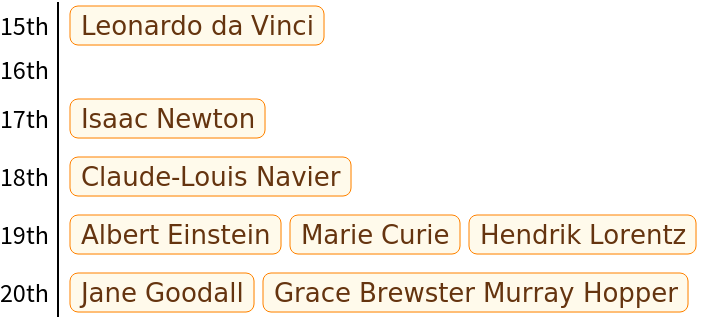Bin some associations based on age:

 In:=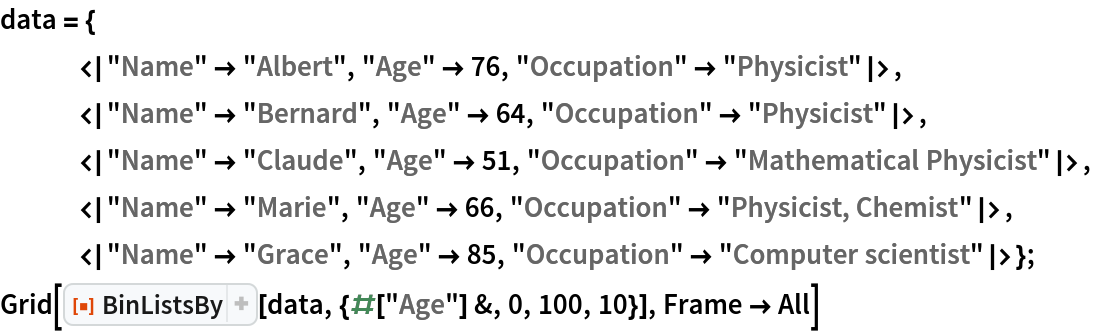Out=### Properties and Relations (2)

BinLists can sometimes perform similar tasks as BinListsBy by giving a single large bin in the "other" dimensions:

 In:=Out=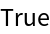GatherBy gives similar output as BinListsBy, but BinListsBy always returns an output with the same dimensions, the bins are sorted, and includes empty lists where necessary:

 In:=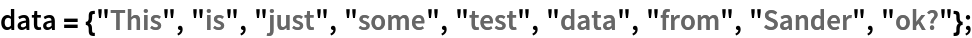In:=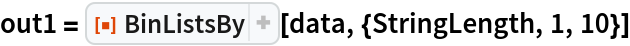Out=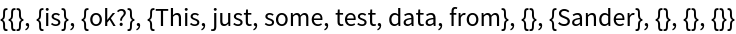In:=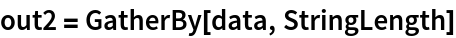Out=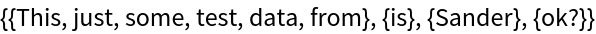Explicitly check they return the same:

 In:=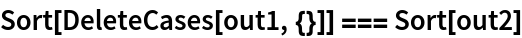Out=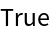### Possible Issues (2)

If the function does not return a number it will not be binned:

 In:=Out=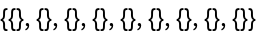Values outside the binning range are discarded:

 In:=Out=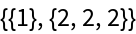### Neat Examples (2)

Bin some datasets based on their regressed slope:

 In:=Out=Plot the datasets with similar slopes together:

 In:=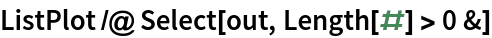Out=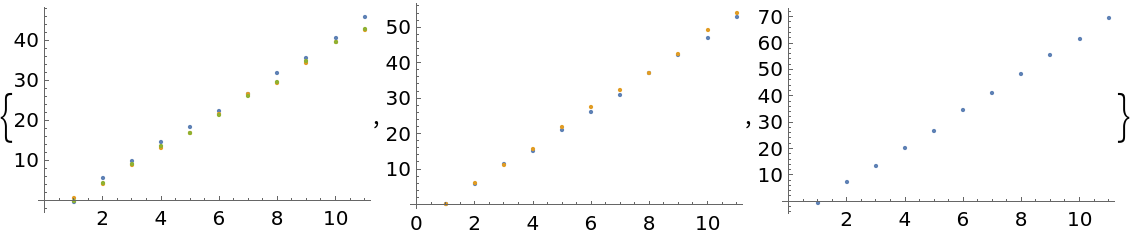SHuisman

## Requirements

Wolfram Language 11.3 (March 2018) or above

## Version History

• 1.0.0 – 01 April 2019